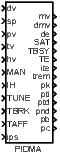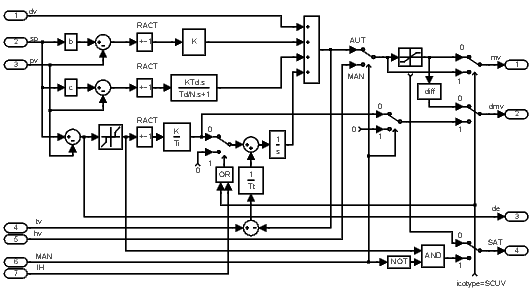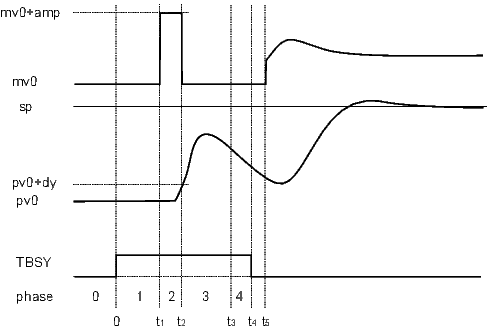### PIDMA – PID controller with moment autotuner

Block SymbolFunction Description
The PIDMA block has the same control function as the PIDU block. Additionally it is equipped with the moment autotuning function.

In the automatic mode ($\mathtt{\text{MAN}}=\mathtt{\text{off}}$), the block PIDMA implements the PID control law with two degrees of freedom in the form

$U\left(s\right)=±K\left\{bW\left(s\right)-Y\left(s\right)+\frac{1}{{T}_{i}s}\left[W\left(s\right)-Y\left(s\right)\right]+\frac{{T}_{d}s}{\frac{{T}_{d}}{N}s+1}\left[cW\left(s\right)-Y\left(s\right)\right]\right\}+Z\left(s\right)$

where $U\left(s\right)$ is Laplace transform of the manipulated variable mv, $W\left(s\right)$ is Laplace transform of the setpoint variable sp, $Y\left(s\right)$ is Laplace transform of the process variable pv, $Z\left(s\right)$ is Laplace transform of the feedforward control variable dv and $K$, ${T}_{i}$, ${T}_{d}$, $N$, $b$ and $c$ are the parameters of the controller. The sign of the right hand side depends on the parameter RACT. The range of the manipulated variable mv (position controller output) is limited by parameters hilim, lolim. The parameter dz determines the dead zone in the integral part of the controller. The integral part of the control law can be switched off and fixed on the current value by the integrator hold input $\mathtt{\text{IH}}=\mathtt{\text{on}}$. For the proper function of the controller it is necessary to connect the output mv of the controller to the controller input tv and properly set the tracking time constant tt.

The rule of thumb for a PID controller is $\mathtt{\text{tt}}\approx \sqrt{{T}_{i}{T}_{d}}$. For a PI controller the formula is $\mathtt{\text{tt}}\approx {T}_{i}∕2$. In this way we obtain the bumpless operation of the controller in the case of the mode switching (manual, automatic) and also the correct operation of the controller when saturation of the output mv occurs (antiwindup).

The additional outputs dmv, de and SAT generate the velocity output (difference of mv), deviation error and saturation flag, respectively.

If the PIDMA block is connected with the block SCUV to configure the 3-point step controller without the positional feedback, then the parameter icotype must be set to 4 and the meaning of the outputs mv and dmv and SAT is modified in the following way: mv and dmv give the PD part and difference of I part of the control law, respectively, and SAT provides the information for the SCUV block whether the deviation error is less than the dead zone dz in the automatic mode. In this case, the setpoint weighting factor c should be zero.

In the manual mode ($\mathtt{\text{MAN}}=\mathtt{\text{on}}$), the input hv is copied to the output mv unless saturated. The overall control function of the PIDMA block is quite clear from the following diagram:The block PIDMA extends the control function of the standard PID controller by the built in autotuning feature. Before start of the autotuner the operator have to reach the steady state of the process at a suitable working point (in manual or automatic mode) and specify the required type of the controller ittype (PI or PID) and other tuning parameters (iainf, DGC, tdg, tn, amp, dy and ispeed). The identification experiment is started by the input TUNE (input TBRK finishes the experiment). In this mode ($\mathtt{\text{TBSY}}=\mathtt{\text{on}}$), first of all the noise and possible drift gradient ($\mathtt{\text{DGC}}=\mathtt{\text{on}}$) are estimated during the user specified time ($\mathtt{\text{tdg}}+\mathtt{\text{tn}}$) and then the rectangle pulse is applied to the input of the process and the first three process moments are identified from the pulse response. The amplitude of the pulse is set by the parameter amp. The pulse is finished when the process variable pv deviates from the steady value more than the dy threshold defines. The threshold is an absolute difference, therefore it is always a positive value. The duration of the tuning experiment depends on the dynamic behavior of the process. The remaining time to the end of the tuning is provided by the output trem.

If the identification experiment is properly finished ($\mathtt{\text{TE}}=\mathtt{\text{off}}$) and the input ips is equal to zero, then the optimal parameters immediately appear on the block outputs pk, pti, ptd, pnd, pb, pc. In the opposite case ($\mathtt{\text{TE}}=\mathtt{\text{on}}$) the output ite specifies the experiment error more closely. Other values of the ips input are reserved for custom specific purposes.

The function of the autotuner is illustrated in the following picture.During the experiment, the output ite indicates the autotuner phases. In the phase of estimation of the response decay rate ($\mathtt{\text{ite}}=\mathtt{\text{-4}}$) the tuning experiment may be finished manually before its regular end. In this case the controller parameters are designed but the potential warning is indicated by setting the output ite=100.

At the end of the experiment (TBSY on$\to$off), the function of the controller depends on the current controller mode. If the $\mathtt{\text{TAFF}}=\mathtt{\text{on}}$ the designed controller parameters are immediately accepted.

Inputs

 dv Feedforward control variable Double (F64) sp Setpoint variable Double (F64) pv Process variable Double (F64) tv Tracking variable Double (F64) hv Manual value Double (F64) MAN Manual or automatic mode Bool off .. Automatic mode on ... Manual mode IH Integrator hold Bool off .. Integration enabled on ... Integration disabled TUNE Start the tuning experiment (off$\to$on) or force transition to the next tuning phase (see the description of the ite output) Bool TBRK Stop the tuning experiment Bool TAFF Tuning affirmation; determines the way the computed parameters are handled Bool off .. Parameters are only computed on ... Parameters are set into the control law ips Meaning of the output signals pk, pti, ptd, pnd, pb and pc Long (I32) 0 .... Designed parameters k, ti, td, nd, b and c of the PID control law 1 .... Process moments: static gain (pk), resident time constant (pti), measure of the system response length (ptd) 2 .... Three-parameter first-order plus dead-time model: static gain (pk), dead-time (pti), time constant (ptd) 3 .... Three-parameter second-order plus dead-time model with double time constant: static gain (pk), dead-time (pti), time constant (ptd) 4 .... Estimated boundaries for manual fine-tuning of the PID controller ($\mathtt{\text{irtype}}=7$) gain k: upper boundary ${\mathtt{\text{k}}}_{hi}$ (pk), lower boundary ${\mathtt{\text{k}}}_{lo}$ (pti) >99 .. Reserved for diagnostic purposes

Outputs

 mv Manipulated variable (controller output) Double (F64) dmv Controller velocity output (difference) Double (F64) de Deviation error Double (F64) SAT Saturation flag Bool off .. The controller implements a linear control law on ... The controller output is saturated TBSY Tuner busy flag Bool TE Tuning error Bool off .. Autotuning successful on ... An error occurred during the experiment ite Error code Long (I32) Tuning error codes (after the experiment): 0 .... No error or waiting for steady state 1 .... Too small pulse getdown threshold 2 .... Too large pulse amplitude 3 .... Steady state condition violation 4 .... Too small pulse aplitude 5 .... Peak search procedure failure 6 .... Output saturation occurred during experiment 7 .... Selected controller type not supported 8 .... Process not monotonous 9 .... Extrapolation failure 10 ... Unexpected values of moments (fatal) 11 ... Abnormal manual termination of tuning 12 ... Wrong direction of manipulated variable 100 .. Manual termination of tuning (warning) Tuning phases codes (during the experiment): 0 .... Steady state reaching before the start of the experiment -1 ... Drift gradient and noise estimation phase -2 ... Pulse generation phase -3 ... Searching the peak of system response -4 ... Estimation of the system response decay rate Remark about terminating the tuning phases TUNE . The rising edge of the TUNE input during the phases -2, -3 and -4 causes the finishing of the current phase and transition to the next one (or finishing the experiment in the phase -4). trem Estimated time to finish the tuning experiment [s] Double (F64) pk Proposed controller gain $K$ ($\mathtt{\text{ips}}=\mathtt{\text{0}}$) Double (F64) pti Proposed integral time constant ${T}_{i}$ ($\mathtt{\text{ips}}=\mathtt{\text{0}}$) Double (F64) ptd Proposed derivative time constant ${T}_{d}$ ($\mathtt{\text{ips}}=\mathtt{\text{0}}$) Double (F64) pnd Proposed derivative component filtering $N$ ($\mathtt{\text{ips}}=\mathtt{\text{0}}$) Double (F64) pb Proposed weighting factor – proportional component ($\mathtt{\text{ips}}=\mathtt{\text{0}}$) Double (F64) pc Proposed weighting factor – derivative component ($\mathtt{\text{ips}}=\mathtt{\text{0}}$) Double (F64)

Parameters

 irtype Controller type (control law)  $\odot$6 Long (I32) 1 .... D 2 .... I 3 .... ID 4 .... P 5 .... PD 6 .... PI 7 .... PID RACT Reverse action flag Bool off .. Higher mv $\to$ higher pv on ... Higher mv $\to$ lower pv k Controller gain $K$. By definition, the value 0 turns the controller off. Negative values are not allowed, use the RACT parameter for such a purpose.  $↓$0.0 $\odot$1.0 Double (F64) ti Integral time constant ${T}_{i}$. The value 0 disables the integrating part (the same effect as disabling it by the irtype parameter).  $↓$0.0 $\odot$4.0 Double (F64) td Derivative time constant ${T}_{d}$. The value 0 disables the derivative part (the same effect as disabling it by the irtype parameter).  $↓$0.0 $\odot$1.0 Double (F64) nd Derivative filtering parameter $N$. The value 0 disables the derivative part (the same effect as disabling it by the irtype parameter).  $↓$0.0 $\odot$10.0 Double (F64) b Setpoint weighting – proportional part  $↓$0.0 $↑$2.0 $\odot$1.0 Double (F64) c Setpoint weighting – derivative part  $↓$0.0 $↑$2.0 Double (F64) tt Tracking time constant. The value 0 stands for an implicit value, which is ${T}_{i}∕2$ or $\sqrt{{T}_{i}{T}_{d}}$ (see above) for controllers with integrating part. For controllers without integrating part, the value 0 disables tracking. If tracking is needed for a P or PD controller, it can be enabled by entering a positive value greater than the sampling time. It is not possible to turn off tracking for controllers with the integrating part (due to the windup effect).  $↓$0.0 $\odot$1.0 Double (F64) hilim Upper limit of the controller output  $\odot$1.0 Double (F64) lolim Lower limit of the controller output  $\odot$-1.0 Double (F64) dz Dead zone Double (F64) icotype Controller output type  $\odot$1 Long (I32) 1 .... Analog output 2 .... Pulse width modulation (PWM) 3 .... Step controller unit with position feedback (SCU) 4 .... Step controller unit without position feedback (SCUV.html) ittype Controller type to be designed  $\odot$6 Long (I32) 6 .... PI controller 7 .... PID controller iainf Type of apriori information  $\odot$1 Long (I32) 1 .... Static process 2 .... Astatic process DGC Drift gradient compensation  $\odot$on Bool off .. Disabled on ... Enabled tdg Drift gradient estimation time [s]  $\odot$60.0 Double (F64) tn Length of noise estimation period [s]  $\odot$5.0 Double (F64) amp Tuning pulse amplitude  $\odot$0.5 Double (F64) dy Tuning pulse get down threshold (absolute difference from the steady pv value)  $↓$0.0 $\odot$0.1 Double (F64) ispeed Desired closed loop speed  $\odot$2 Long (I32) 1 .... Slow closed loop 2 .... Normal (middle fast) closed loop 3 .... Fast closed loop ipid PID controller form  $\odot$1 Long (I32) 1 .... Parallel form 2 .... Series form

The PIDMA block is available in REXYGEN.